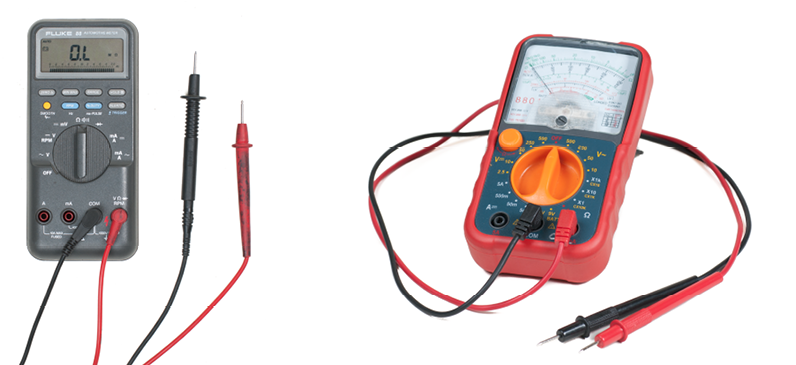# 13.1.1: Describe the use and storage of meters

•• Camosun College
$$\newcommand{\vecs}{\overset { \rightharpoonup} {\mathbf{#1}} }$$ $$\newcommand{\vecd}{\overset{-\!-\!\rightharpoonup}{\vphantom{a}\smash {#1}}}$$$$\newcommand{\id}{\mathrm{id}}$$ $$\newcommand{\Span}{\mathrm{span}}$$ $$\newcommand{\kernel}{\mathrm{null}\,}$$ $$\newcommand{\range}{\mathrm{range}\,}$$ $$\newcommand{\RealPart}{\mathrm{Re}}$$ $$\newcommand{\ImaginaryPart}{\mathrm{Im}}$$ $$\newcommand{\Argument}{\mathrm{Arg}}$$ $$\newcommand{\norm}{\| #1 \|}$$ $$\newcommand{\inner}{\langle #1, #2 \rangle}$$ $$\newcommand{\Span}{\mathrm{span}}$$ $$\newcommand{\id}{\mathrm{id}}$$ $$\newcommand{\Span}{\mathrm{span}}$$ $$\newcommand{\kernel}{\mathrm{null}\,}$$ $$\newcommand{\range}{\mathrm{range}\,}$$ $$\newcommand{\RealPart}{\mathrm{Re}}$$ $$\newcommand{\ImaginaryPart}{\mathrm{Im}}$$ $$\newcommand{\Argument}{\mathrm{Arg}}$$ $$\newcommand{\norm}{\| #1 \|}$$ $$\newcommand{\inner}{\langle #1, #2 \rangle}$$ $$\newcommand{\Span}{\mathrm{span}}$$$$\newcommand{\AA}{\unicode[.8,0]{x212B}}$$

## Describe the use and storage of meters

A technician is only as accurate as the measurement equipment being used. If the equipment is misused or is faulty, then the measurements will be inaccurate. If the measurements are inaccurate, then the technician will draw the wrong conclusions. To avoid getting inaccurate readings, you need to handle, use, and store meters properly.

The two major types of meters are digital and analog (Figure 1). Although both meters perform the same functions, they look different. As you can see, the difference is in the display unit. Digital meters are usually simpler to use and are more accurate than analog meters, and therefore have become more popular. We will focus on the digital multimeter (DMM), as it is the most common type in use, although analog multimeters may still be preferable in some cases, for example, when monitoring a rapidly varying value.Figure $$\PageIndex{1}$$: Digital and analog multimeters (CC BY-NC-SA; BC Industry Training Authority)